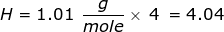# STP Formula

STP Formula

STP is a shortened version of the words standard temperature and pressure. Standard temperature and pressure are defined as 0°Celsius, and 1.00 atmospheres, respectively. Avogadro determined that the volume of any gas measured at STP is 22.4 L.This value is used in many gas conversions from liters to moles, or vice-versa, as long as the STP conditions are met. It is key to remember that the STP value of 22.4 L can only be considered for gaseous particles.

STP Formula Questions:

1. How many liters does 3.7 moles of oxygen gas (O2) at STP occupy?

Answer:2. How many liters does 45.3 grams of methane gas (CH4) at STP occupy?

Answer:

This problem requires that the grams of methane gas be converted into moles before using Avogadro's value.

First, find the molar mass of CH4.Now, set up the problem and solve.Related Links: